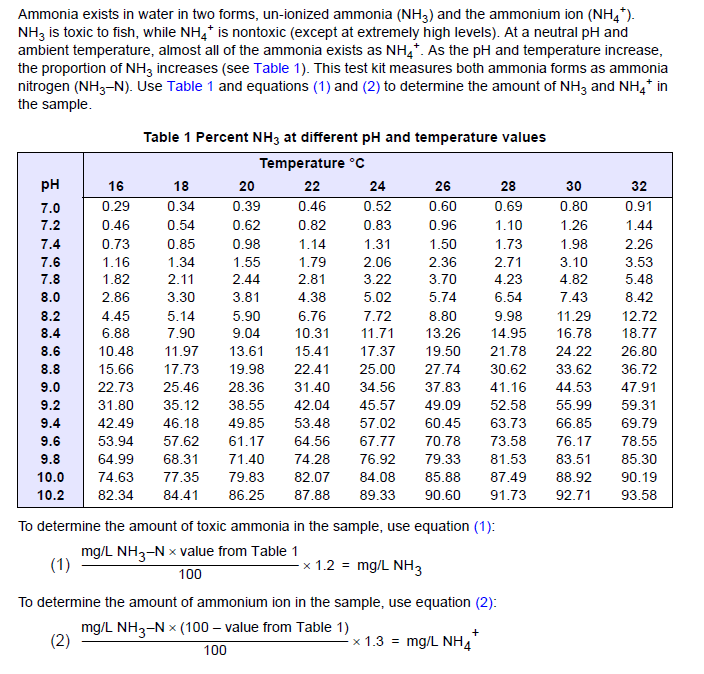﻿

# Do the Nessler and Salicylate methods detect ammonia or ammonium?

## Document ID

Document ID TE929

## Published Date

Published Date 08/19/2020
Question
Do the Nessler and Salicylate methods detect ammonia or ammonium?
Summary
Detection of ammonia and ammonium using Nessler and Salicylate methods.
Both the Nessler and Salicylate methods have a reaction with molecular ammonia in a basic solution. If the original sample contained ammonium ions, the basic reagents would convert it to molecular ammonia, and then it will react and contribute to the final test result. Therefore, the term “total ammonia” can be used. However, these simple ammonia tests do not include the ammonia that is in organically-bonded amino groups. A true total ammonia test would require heating the sample in an acidic solution to digest the organic ammonia. An ammonia test and then following with a test for ammonia.

Since the test result is a sum of both molecular ammonia (NH 3) and ionic ammonium (NH 4 +), the preferred unit for reporting is as nitrogen (NH 3-N). Most Hach colorimeters and spectrophotometers have the option to convert the test results between nitrogen, ammonia, and ammonium. This does not change the chemistry of the test, it only changes the units. This is similar to reporting height in inches, centimeters, or meters. The units change, but not the measurement. It should be noted that the stoichiometric ratio of N to NH 3 and NH 4+ are not exactly equal:

• To convert from mg/L NH 3-N to mg/L NH 3, multiply by 1.216
• Calculated from the mass of NH 3 divided by the mass of N (17.034 ÷ 14.01 = 1.216)
• To convert from mg/L NH 3-N to mg/L NH 4, multiply by 1.288
• Calculated from the mass of NH 4 + divided by the mass of N (18.042 ÷ 14.01 = 1.288)

After conducting an ammonia test using Nessler or Salicylate chemistry (the reported results are the sum of NH 3 and NH 4 +), it may be important to differentiate the amount of molecular ammonia from ammonium ions. The table, which is attached to this article, relates the percentage of molecular ammonia to pH and temperature. Under the pH/temperature chart, equations used to calculate ammonia and ammonium are included.